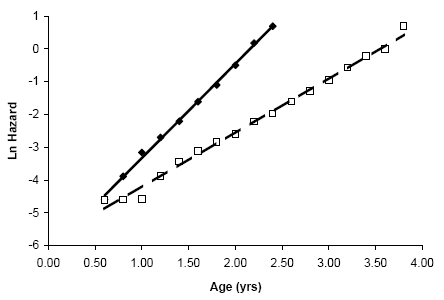# Estimating the Rate of Ageing

The rate of ageing of a given population can be quantified by calculating the slope of the mortality curve of the population. In fact, determining whether the slope of the mortality curve was altered is one method that allows scientists to estimate whether the rate of ageing changed between two different cohorts, such as two cohorts exposed to different conditions or from different genetic backgrounds. As an example, below are two hypothetical mouse cohorts. Because the slope of the mortality curve changes in the experimental conditions when compared to the controls this would suggest that the basic rate of ageing was altered by the treatment. As described below, it is also possible to determine statistically whether the slope of the curve changed or not.Legend: natural logarithm of mortality curves of two hypothetical two mouse cohorts. Lines represent estimated adult mortality trajectories based on the Gompertz parameters for controls (straight line) and for a cohort subjected to a given treatment (dashed line).

The figure above employs the Gompertz curve. Though other mathematical functions can also be used, the Gompertz function is arguably the simplest function and is given by:

m(t) = AeGt

Where m(t) is the mortality rate as a function of time or age (t), A is the extrapolated constant to birth or maturity, and G is the exponential (Gompertz) mortality rate coefficient. By regression analysis, which should normally only encompass the period after the mortality rate begins to climb, it is possible to estimate the Gompertz equation for both cohorts in the figure: m(t) = 0.0022e2.82t for controls and m(t) = 0.0029e1.64t for the treated animals. From the Gompertz equation we can obtain the mortality rate doubling time (MRDT), which is estimated from the slope of the curve by:

MRDT = 0.693/G

As such, the MRDT is considered to be a measure of the rate of ageing. In the above example, the MRDT for the controls is 0.693/2.82 = 0.25 years; in the treated animals it is 0.693/1.64 = 0.42. In this case, it appears that the treatment altered the demographic rate of ageing.

Another parameter used to infer the ageing patterns of a population is the initial mortality rate (IMR), which represents the mortality rate independently of the ageing process. Though there are different methods, the IMR is typically estimated from the mortality rate prior to its exponential increase with age, which can be obtained from the mortality curve (by looking at the baseline mortality prior to its exponential increase) or, for mice, by using t = 0.25 in the Gompertz equation. For the example above, the IMR = 0.0022 * e2.82 * 0.25 = 0.0045 for the controls and IMR = 0.0044 for the treated animals.

The MRDT and IMR can also be used to compare rates of ageing between different species and a few values are given in the AnAge database.

## SPSS Script and Example Dataset

In order to make the above calculations, you can download the SPSS syntax we generally use. The syntax was tested in various SPSS versions from 11.5 until 22.0, and it should work with later versions. It is divided into self-explanatory sections. Please read the syntax prior to running it and make sure you understand its different sections. The syntax will not work if you just run it because it is meant to be run in parts and you need to have some data first.

mortality.txt is the data used to generate the figure and equations above. Sample 1 is the controls and sample 2 is the treated animals. qx is the age-specific mortality estimated as the number of animals alive at the end of the interval over the number of animals at the start of the interval. The hazard rate, from which the Gompertz parameters are estimated, can be estimated with the SPSS syntax and is given by 2qx/(2 - qx). You may use this data together with the SPSS syntax as an exercise.

## Citation, Disclaimer and Copyright

The method described above is further detailed, discussed, and applied in:

de Magalhaes, JP et al. (2005) "The influence of genes on the aging process of mice: a statistical assessment of the genetics of aging" Genetics 169:265-274.

de Magalhaes, JP et al. (2018) "A reassessment of genes modulating aging in mice using demographic measurements of the rate of aging" Genetics 208:1617-1630.

If the information on this page is helpful for your work or if you employ our SPSS syntax, please cite the above papers in any subsequence publications, presentations, etc.

We are not responsible for any damage the use of this SPSS syntax may cause. Use at your own risk. Copyright © 2004 - 2007, 2018 by João Pedro de Magalhães. You may distribute this SPSS syntax according to the same conditions of the GNU public licence.